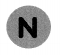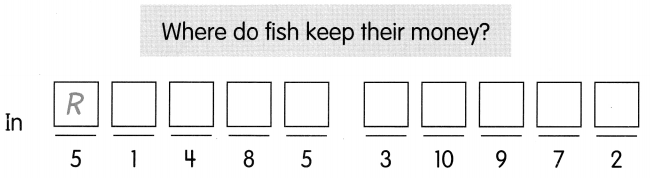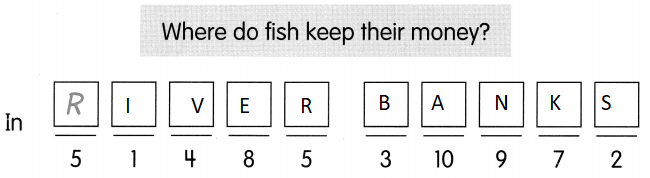# Math in Focus Grade 1 Chapter 4 Practice 2 Answer Key Ways to Subtract

This handy Math in Focus Grade 1 Workbook Answer Key Chapter 4 Practice 2 Ways to Subtract detailed solutions for the textbook questions.

## Math in Focus Grade 1 Chapter 4 Practice 2 Answer Key Ways to Subtract

Fill in each number bond. Then complete the subtraction sentence.

Example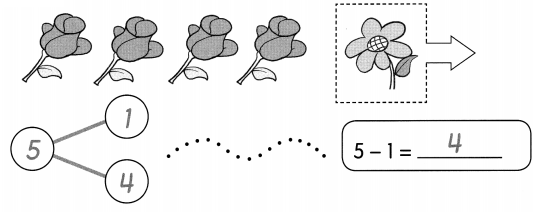Question 1.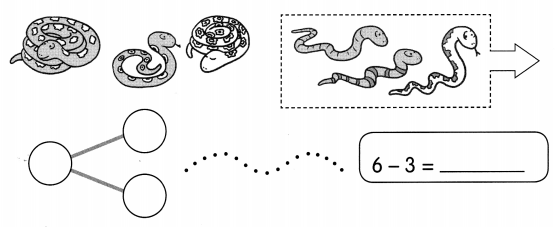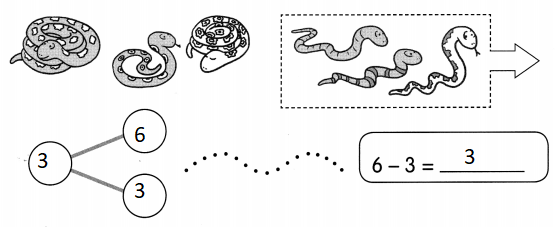Explanation:
6 – 3 = 3
The difference of 6 and 3 is 3

Question 2.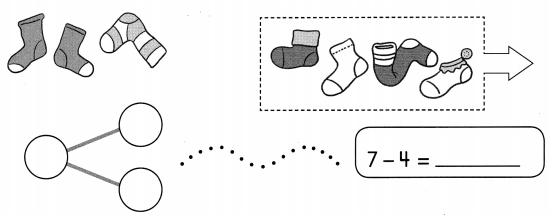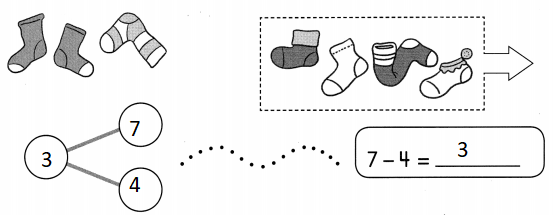Explanation:
7 – 4 = 3
The difference of 7 and 4 is 3

Question 3.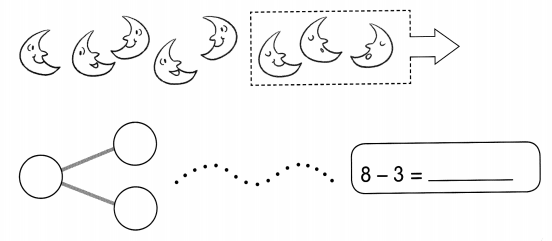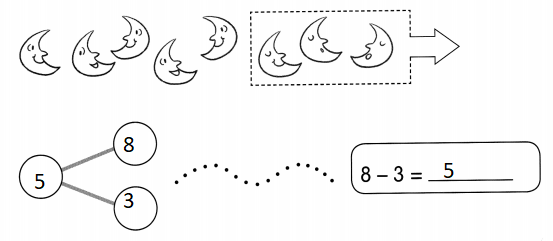Explanation:
The difference of 8 and 3 is 5

Question 4.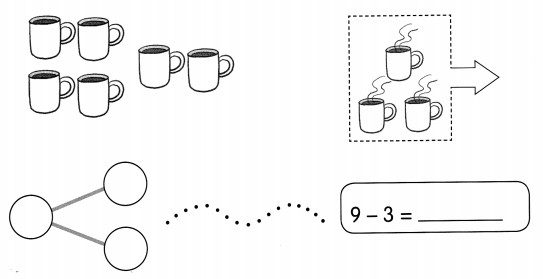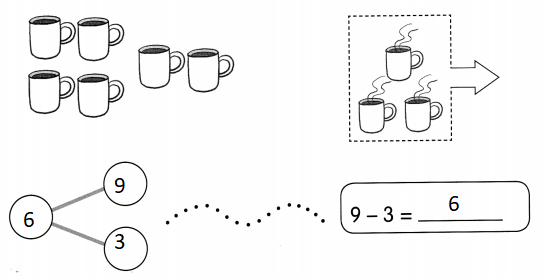Explanation:
9 – 3 = 6
The difference of 9 and 3 is 6

Question 5.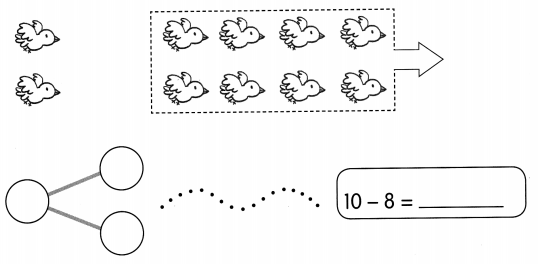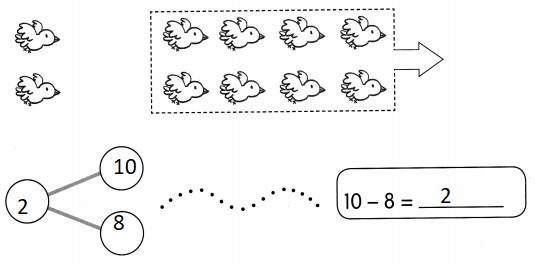Explanation:
10 – 8 = 2
The difference of 10 and 8 is 2

Fill in the number bonds.
Then write the missing numbers in the subtraction sentences.

Example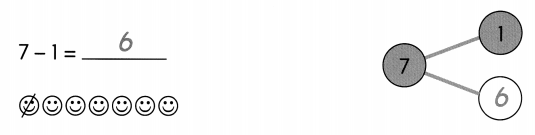Question 6.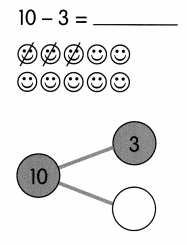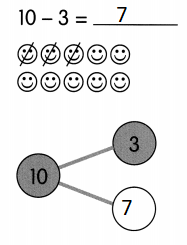Explanation:
The difference of 10 and 3 is 7
10 – 3 = 7
Explained with number bond.

Question 7.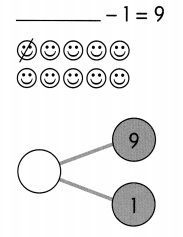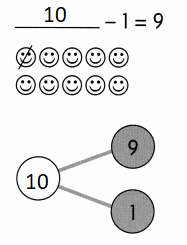Explanation:
10 – 1 = 9
The difference of 10 and 1 is 9
Explained with number bond.

Question 8.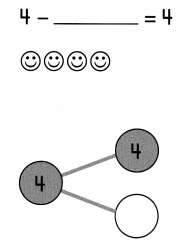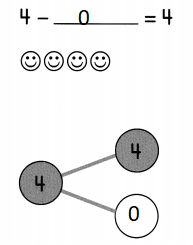Explanation:
4 – 0 = 4
The difference of 4 and 0 is 4
Explained with number bond.

Question 9.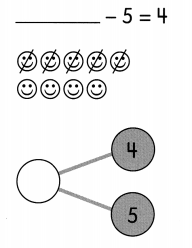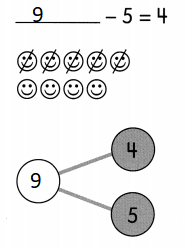Explanation:
9 – 5 = 4
The difference of 9 and 5 is 4
Explained with number bond.

Some slickers are torn off. Write a subtraction sentence to find how many are left.

Example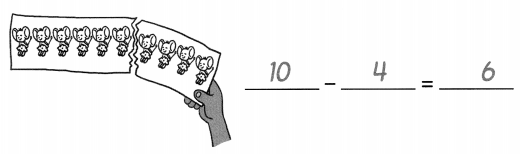Question 10.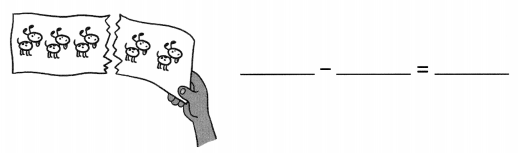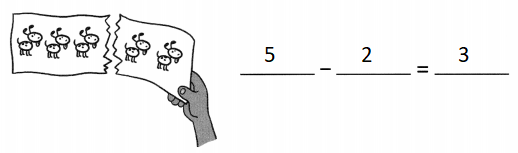Explanation:
5 – 2 = 3
The difference of 5 and 2 is 3

Question 11.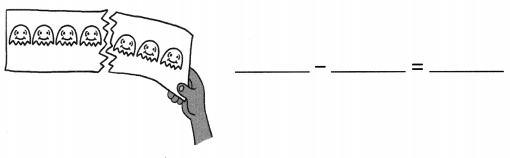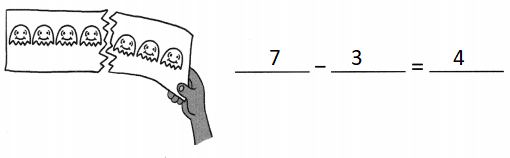Explanation:
7 – 3 = 4
The difference of 7 and 3 is 4

Question 12.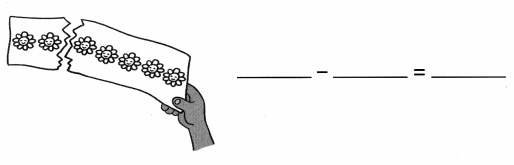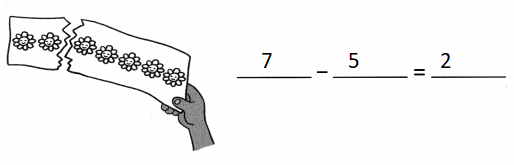Explanation:
7 – 5 = 2
The difference of 7 and 5 is 2

Subtract. Then match the answers to show where each animal lives.

Question 13.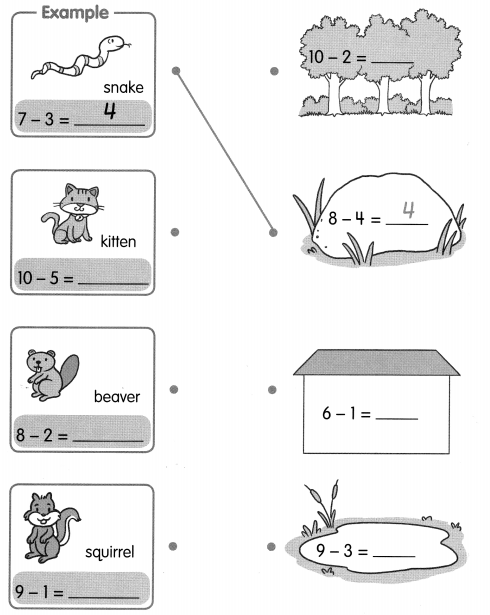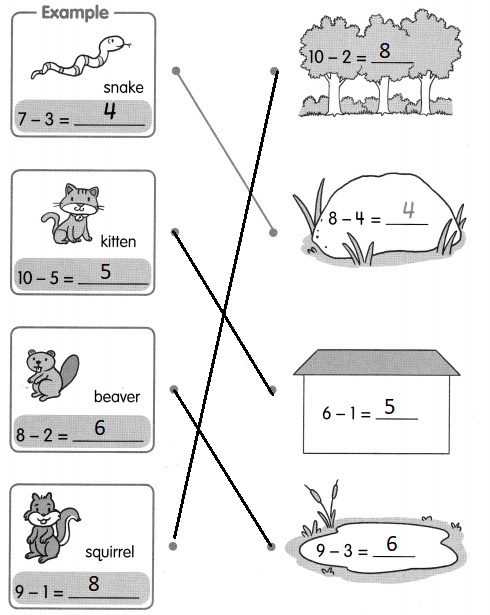Explanation:
Matched the subtraction sentences with the subtraction facts.

Complete.
Then write the letters in the correct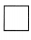to solve the riddle.

Question 14.
10 – 5 = 5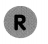Explanation:
10 – 5 = 5
The difference of 10 and 5 is 5

Question 15.
9 – 8 = ____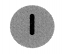Explanation:
9 – 8 = 1
The difference of 9 and 8 is 1

Question 16.
6 – 3 = ______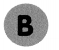Explanation:
6 – 3 = 3
The difference of 6 and 3 is 3

Question 17.
7 – 5 = ____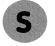Explanation:
7 – 5 = 2
The difference of 7 and 5 is 2
7 – 5 = 2

Question 18.
9 – 4 = ___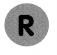Explanation:
9 – 4 = 5
The difference of 9 and 4 is 5

Question 19.
10 – 0 = ______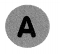Explanation:
10 – 0 = 10
The difference of 10 and 0 is 10

Question 20.
9 – 1 = ______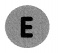Explanation:
9 – 1 = 8
The difference of 9 and 1 is 8

Question 21.
6 – 2 = ______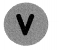Explanation:
6 – 2 = 4
The difference of 6 and 2 is 4

Question 22.
10 – 3 = ____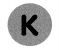Explanation:
10 – 3 = 7
The difference of 10 and 3 is 7

Question 23.
9 – 0 = ______